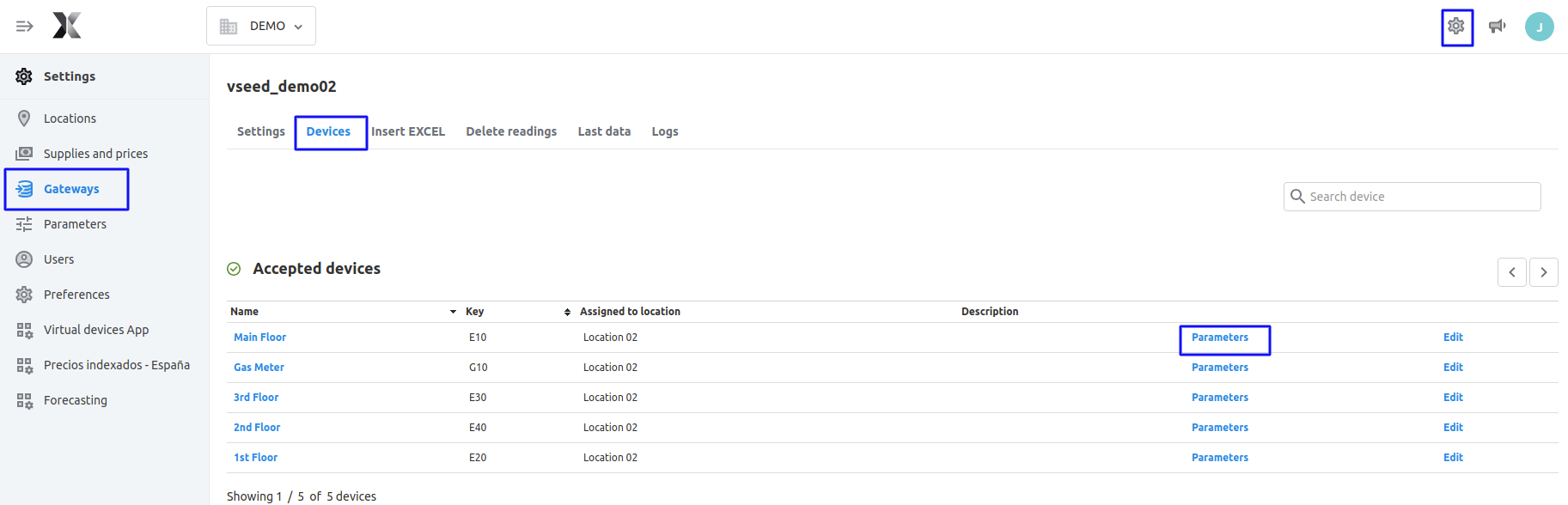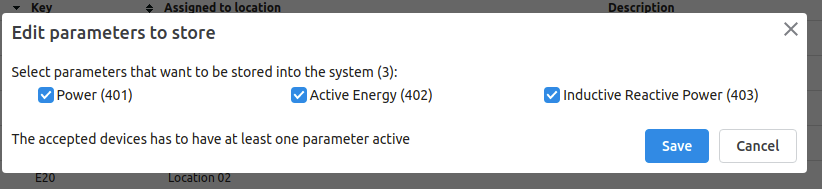# Introduction to Parameters

This article includes a description of the parameters that can be inserted into the platform, as well as the methodology that should be followed depending on the type of variable that wants to be inserted. Moreover, the algorithm that the software follows in order to calculate all the data frequencies, will be summarised. Finally, some additional information will be provided in order to solve possible problems that might arise.

The information will be divided into the following sections:

### Parameter definition

A parameter is what allows the platform to understand which type of consumption or variable a device is monitoring. It is really important to correctly define a parameter, as alerts, visualisation of the values and other functionalities will depend on it.

It is important to understand that one device can have a lot of parameters but each of them must be unique. This means that you may have 15 parameters in one device but all of them must be distinct. Then, if a gateway sends data from 2 gas sensors, in our platform this could not be represented by one device only, as it would cause the parameter gas to be repeated. Thus, each sensor should represent a different device, and both devices would then have the parameter gas.

### Available parameters in the platform

Multiple parameters are accepted in the platform. The list of all the available parameters can be found in the following spreadsheet:

As it can be noticed, these parameters include both discrete and continuous variables. All of them are here represented by a code of 3 digits. However, when inserting data, depending on the type of variable that is being inserted, some more digits may be necessary.Please go to section 3 to learn more about the insertion of parameters into the platform and the available frequencies for each of them.

### Inserting parameters into the platform - Available frequencies

In order to understand how a parameter can be inserted into the platform, there needs to be a differentiation between discrete and continuous variables.

Discrete variables, such as temperature or power, are usually inserted using the 3-digits number shown in the excel file of the previous section: "Available parameters in the platform"

Continuous variables, such as consumed electricity and gas, can be inserted in two different ways in the platform:

1. Basic parameter. The basic methodology implies data inserted cumulatively, i.e. every measure will be added to the previous one. This results in constantly increasing values and creates a link between the measures. This methodology is frequency-neutral, as it does not directly relate to a certain insertion frequency.
2. Discrete parameter. The discrete methodology implies that every measure will directly be uploaded into the platform, despite any prior measures. Every measure inserted into the platform will be independent of the others. This methodology directly relates to a specific insertion frequency.

#### Inserting with the basic methodology

While inserting through the basic methodology, it is not necessary to indicate to the software which is the frequency in which data is being uploaded, as the platform itself will identify it.

If data will be inserted through the basic methodology, the representation of each parameter will be the 3 digits that are represented in the excel file of the section:  "Available parameters in the platform".

When inserting data using this methodology, the software will carry out several calculations so as to determine the specific consumption for different frequencies. Please go to "Calculations carried out after the insertion" in order to learn more about these calculations.

#### Inserting with the discrete methodology

While inserting through the discrete methodology, it is necessary to specify to the software which is the frequency in which the data is being uploaded. Moreover, an "operation" needs to be defined so as to understand the type of variable that is being monitored.

The code for discrete values' insertion is SSSFO, in which:

• SSS corresponds to the 3-digits representing the parameter, found in the excel file of section "Available parameters in the platform".
• F represents the frequency in which data is being inserted:
 Frequency F value Quarter-hourly 0 Hourly 1 Daily 2 Weekly 3 Monthly 4 Yearly 5
• O represents the operation that will determine how the data has been discretised:
 Operation O value Discretised 1 Average 2 Maximum 3 Minimum 4 One-on-one 5

Operation values from 1 to 4 are usually used for continuous variables, such as electricity or water consumption. In these cases, the operation would mean if the inserted discrete value represents the consumed electricity, the average, the maximum or the minimum; Operation values 2 to 5 are usually used for discrete variables such as temperature or power. Note that operation 5 would represent an instantaneous value, different from sending average values of the variables during a certain period of time.

### Calculations carried out after the insertion

Before understanding the calculations that the platform carries out once a parameter has been introduced, it is needed to know the available frequencies that can be found in the platform:
• 5 minutes.
• 10 minutes.
• Quarter-hourly.
• Half-hourly.
• Hourly.
• Daily.
• Weekly.
• Monthly.
• Yearly.

#### Calculations for discrete data insertion

Discrete data insertion can be directly displayed in the analysis section in the frequency it has been inserted. This means that the user will visualise exactly the same value they have inserted.

Imagine that data is inserted every half hour, and a value of 15 kWh is uploaded at 10:00h and a value of 20 kWh at 10:30h. Then, the hourly consumption could be calculated by adding the consumption of the two half-hourly introduced values, i.e. 35 kWh. If all the values of the day were uploaded, the same could be calculated for the daily frequency, and so on.

This procedure is exactly the one followed by the platform. Once a parameter has been introduced following a certain frequency, all the smaller frequencies will be automatically calculated, i.e. if data is introduced every day: the weekly, monthly and yearly frequencies will be calculated. However, all the higher frequencies will remain blank.

Note: If data is inserted in a weekly resolution, the monthly resolution will not be calculated. This is due to the fact that months do not always start on the first day of the week, nor end on the last day of a week, and thus adding weekly calculations does not fully cover the monthly consumption in most cases. In order to not interpolate, the monthly data is not calculated, to be coherent with how the platform calculates lower resolution data.

#### Calculations for basic data insertion

Basic data insertion cannot be directly displayed in the analysis screen, as the inserted data will represent cumulative values. Therefore, the platform will interpolate between values to identify the consumption between them.

As an example, imagine that the consumption of 1000 kWh is uploaded at 10:00h; Then, the consumption of 1100 kWh is uploaded at 11:00h. It is known that the consumption between 10:00h and 11:00h has been of 100 kWh, so the hourly consumption displayed in the analysis screen at 10:00h will be of 100 kWh. Moreover, it can also be estimated that the quarter-hourly consumption between 10:00h and 11:00h is of 25 kWh, so the quarter-hourly consumption displayed in the analysis screen at 10:00h, 10:15h, 10:30h and 10:45h will be of 25 kWh.

As a conclusion, if data is introduced following the basic methodology, all frequencies will be automatically calculated, following a simple interpolation as shown in the previous examples.

### Management of the inserted parameters in the platform

Once the data has been introduced into the platform, in order to see and manage the parameters that are being introduced by the devices, you should go to Settings > Gateways (select a gateway and click to edit) > Devices > Parameters:Once you click on Parameters, you will see the following window:In this menu, you will be able to select and de-select all the parameters that have been received by your device.

In case you observe you are receiving a parameter that is not useful to analyse, you have two options:

1. De-select this parameter, and it will no longer cost a datapoint to you.
2. Derive the parameter into a more useful one, e.g. from pulses to gas volume or energy.

### Visualisation of the parameters

Once all the parameters have been introduced into the platform and have been accepted by the user, it is time to visualise them in the analysis screens. Please find below a table with the parameters that can be visualised in every screen:

 Screen/Parameter Energy consumption* Temperature Cost Others** Consumption x - - - Cost x - x - Comparison x - - - Comfort - x - - Peak demand x - - - By device x x x x Advanced Analytics x x x x Queries x x x x

*Energy consumption refers to:

• Electricity consumption
• Gas (kWh, volume and normalised volume)
• Water
• Diesel (kWh and volume)
• Produced electricity
• Thermal energy (heating and cooling)
• Compressed air volume
• Liquified natural gas
• Biomass

**Please note that Power is included in the category "Others", together with Voltage and Current.